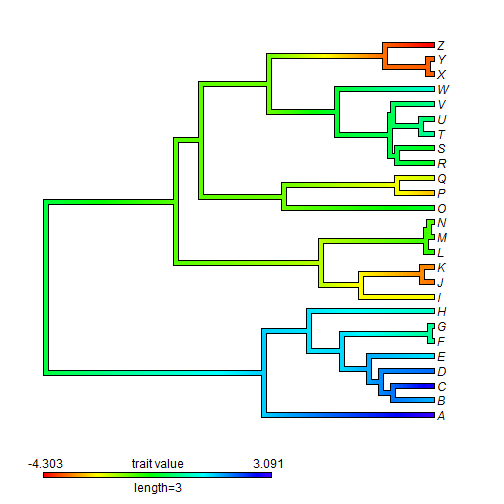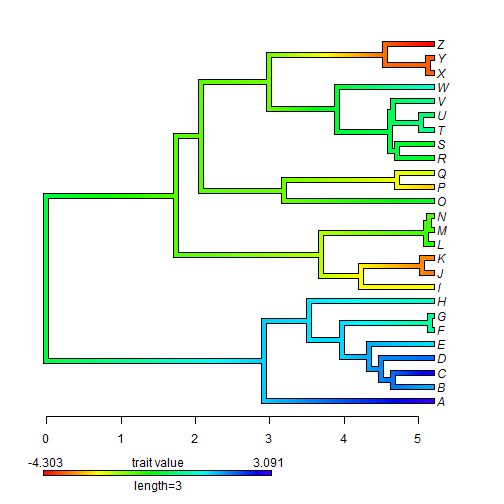## Friday, June 30, 2017

### Plotting a legend outside the plot area using `add.color.bar` (& in general)

Recently I received the following question:

“I am using the add.color.bar function to add a colorbar to an existing plot.
Do you know if it is possible to move the added colorbar to the outside of the plot domain? Do you think I would need to modify code in the actual function?”

Evidently, this can be done using `par(xpd=TRUE)`. For instance:

``````library(phytools)
tree
``````
``````##
## Phylogenetic tree with 26 tips and 25 internal nodes.
##
## Tip labels:
##  A, B, C, D, E, F, ...
##
## Rooted; includes branch lengths.
``````
``````x
``````
``````##           A           B           C           D           E           F
##  3.09148522  1.54096027  2.91508379  2.00469068  1.15813551  0.29543463
##           G           H           I           J           K           L
##  0.27951056  0.60805259 -2.52405841 -3.50891295 -3.34674083 -0.97428407
##           M           N           O           P           Q           R
## -1.25336677 -1.40812351 -0.51371266 -2.92582374 -2.24608676 -0.53203275
##           S           T           U           V           W           X
## -0.59487179  0.30811419 -0.08364322  0.16895725  0.70189973 -3.64724619
##           Y           Z
## -3.65517946 -4.30271550
``````
``````obj<-contMap(tree,x,plot=FALSE)
plot(obj,legend=FALSE,mar=c(5.1,2.1,2.1,2.1))
par(xpd=TRUE)
add.color.bar(leg=3,cols=obj\$cols,title="trait value",lims=obj\$lims,
digits=3,prompt=FALSE,x=0,y=-3)
``````We can easily see that this is outside of our plotting domain by adding axis labels to the plot as follows:

``````plot(obj,legend=FALSE,mar=c(6.1,2.1,2.1,2.1))
par(xpd=TRUE)
add.color.bar(leg=3,cols=obj\$cols,title="trait value",lims=obj\$lims,
digits=3,prompt=FALSE,x=0,y=-4)
axis(1)
``````You get the general idea.

#### Post a Comment

Note: due to the very large amount of spam, all comments are now automatically submitted for moderation.